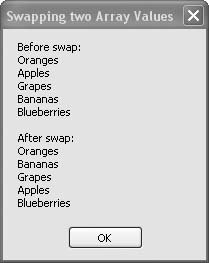# Recipe 8.6. Swapping Two Array Values

#### Problem

You want to swap the contents of any two elements in an array.

#### Solution

Sample code folder: Chapter 08\SwapArrayElements

Write a custom method that reverses the positions of two specific array elements. The code in this recipe implements a Swap() method that does just that.

#### Discussion

The Swap() method accepts an array of any data type, plus the positions of two elements to swap. After doing some boundary checking, it reverses the elements:

` Public Sub Swap(ByRef swapArray( ) As Object, _       ByVal first As Integer, ByVal second As Integer)    ' ----- Reverse two elements of an array.    Dim tempObject As Object    ' ----- Check for invalid positions.    If (first < 0) Then Return    If (first >= swapArray.Length) Then Return    If (second < 0) Then Return    If (second >= swapArray.Length) Then Return    If (first = second) Then Return    ' ----- Reverse two elements.    tempObject = swapArray(first)    swapArray(first) = swapArray(second)    swapArray(second) = tempObject End Sub `

Several lines of this code simply check to make sure the indexes into the array are valid. If they are out of range, no swapping takes place.

The following code demonstrates the Swap() method by creating a string array of fruit names and swapping the contents at the first and third indexes into the array. The ArrayHelper is instanced to accept string parameters, and the string array is passed to its Swap() method:

` Dim result As New System.Text.StringBuilder Dim arraySwap( ) As String = { _    "Oranges", "Apples", "Grapes", "Bananas", "Blueberries"} ' ----- Show the pre-swap data. result.AppendLine("Before swap:") For Each fruit As String In arraySwap     result.AppendLine(fruit) Next fruit ' ----- Swap two elements. Swap(arraySwap, 1, 3) ' ----- Show the post-swap data. result.AppendLine( ) result.AppendLine("After swap:") For Each fruit As String In arraySwap     result.AppendLine(fruit) Next fruit MsgBox(result.ToString( )) `

Figure 8-6 shows the array's contents before and after elements 1 and 3 are swapped. Notice that the array elements start at zero, so the swap is between the second and fourth values in the array.

##### Figure 8-6. Swapping two array elements with the Swap( ) methodRecipe 8.5 shows how to randomly rearrange the contents of an entire array.Visual Basic 2005 Cookbook: Solutions for VB 2005 Programmers (Cookbooks (OReilly))
ISBN: 0596101775
EAN: 2147483647
Year: 2006
Pages: 400

Similar book on Amazon